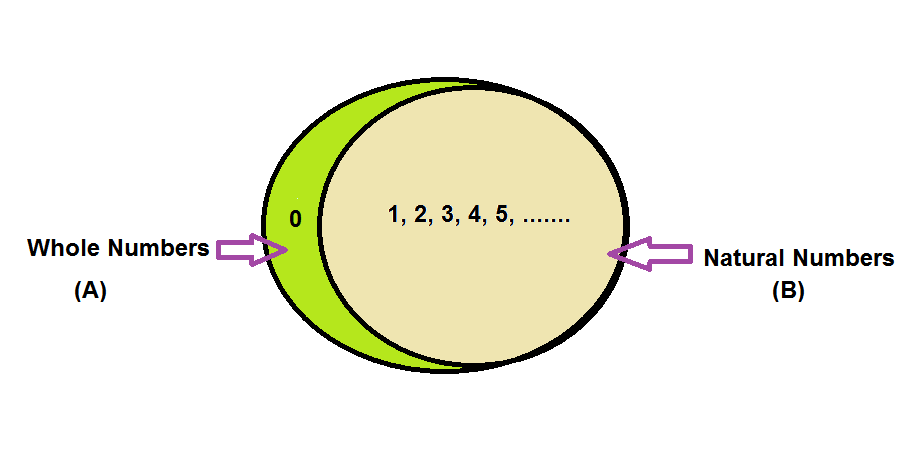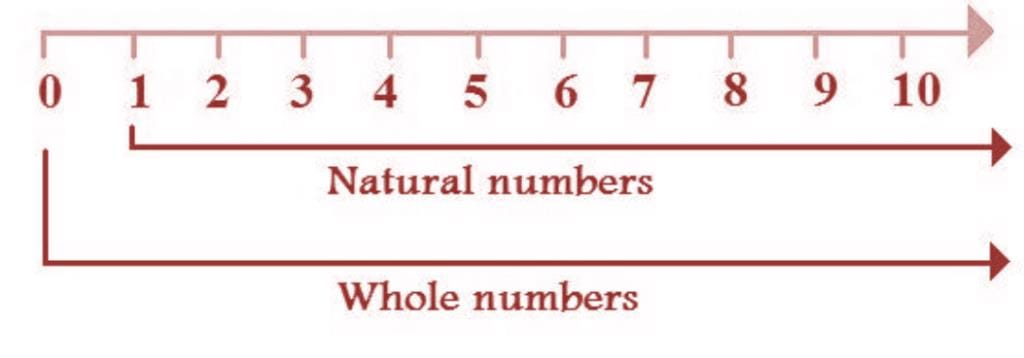# Natural Numbers Notes - Class 6

## Class 6: Natural Numbers Notes - Class 6

The document Natural Numbers Notes - Class 6 is a part of Class 6 category.
All you need of Class 6 at this link: Class 6

Natural numbers are a part of the number system which includes all the positive integers from 1 till infinity. It should be noted that the natural numbers include only the positive integers i.e. set of all the counting numbers like 1, 2, 3, ………. excluding the fractions, decimals, and negative numbers. In this article, we are going to learn more about natural number with respect to its definition, comparison with whole numbers, representation in the number line, properties, etc.

## Natural Number Definition

As explained in the introduction part, Natural numbers are the numbers which are positive in nature and includes numbers from 1 till infinity(∞). These numbers are countable and are usually used for calculations purpose.  The set of natural numbers are represented by the letter “N”.

## Natural Numbers and Whole Numbers

Natural numbers include all the whole numbers excluding the number 0. In other words, all natural numbers are whole numbers, but all whole numbers are not natural numbers. Check out the difference between natural and whole numbers to know more about the differentiating properties of these two sets of numbers.The above representation of sets shows two regions,

A ∩ B ie. intersection of natural numbers and whole numbers (1, 2, 3, 4, 5, 6, ……..) and the green region showing A-B, i.e. part of the whole number (0).

Thus, a whole number is “a part of Integers consisting of all the Natural number including 0.”

### Is ‘0’ a Natural Number?

The answer to this question is ‘No’. As we know already, natural numbers start with 1 to infinity and are positive in nature. But when we mention 0 with a positive integer such as 10, 20, etc. it becomes a natural number. In fact, 0 is a whole number which has a null value.

### Representing Natural Numbers on a Number Line

Natural numbers representation on a number line is as follows:The above number line represents natural numbers and the whole numbers on a number line. All the integers on the right-hand side of 0 represent the natural numbers, thus forming an infinite set of numbers. When 0 is included, these numbers become whole numbers which are also an infinite set of numbers.

### Set of Natural Numbers

In set notation, the symbol of natural number is “N” and it is represented as given below.

Statement:

N = Set of all numbers starting from 1.

In Roster Form:

N = {1, 2, 3, 4, 5, 6, 7, 8, 9, 10, ………………………………}

In Set Builder Form:

N = {x : x is an integer starting from 1}

### Natural Numbers Examples

The natural numbers include the positive integers (also known as non-negative integers) and a few examples include 1, 2, 3, 4, 5, 6, ..∞.  In other words, natural numbers are a set of all the whole numbers excluding 0.

### Properties of Natural Numbers

Natural numbers properties are segregated into four main properties which include closure property, commutative property, associative property, and distributive. Each of these properties is explained below in detail.

#### Closure Property

The natural numbers are always closed under addition and multiplication i.e. the addition and multiplication of natural numbers will always yield a natural number. In the case of subtraction and division, natural numbers are not closed which means subtracting or dividing two natural numbers might not give a natural number as a result.

• Addition: 1 + 2 = 3, 3 + 4 = 7, etc. In each of these cases, the resulting number is alwasy a natural number.
• Multiplication: 2 × 3 = 6, 5 × 4 = 20, etc. In this case also, the resultant is always a natural number.
• Subtraction: 9 – 5 = 4, 3 – 5 = -2, etc. In this case, the result may or may not be a natural number.
• Division: 10 ÷ 5 = 2, 10 ÷ 3 = 3.33, etc. In this case also, the resultant number may or may not be a natural number.

#### Associative Property

The associative property holds true in case of addition and multiplication of natural numbers i.e. a + ( b + c ) = ( a + b ) + c and a × ( b × c ) = ( a × b ) × c. On the other hand, for subtraction and division of natural numbers, the associative property does not hold true. An example of this is given below.

• Addition: a + ( b + c ) = ( a + b ) + c => 3 + (15 + 1 ) = 19 and (3 + 15 ) + 1 = 19.
• Multiplication: a × ( b × c ) = ( a × b ) × c => 3 × (15 × 1 ) = 45 and ( 3 × 15 ) × 1 = 45.
• Subtraction: a – ( b – c ) ≠ ( a – b ) – c => 2 – (15 – 1 ) = – 12 and ( 2 – 15 ) – 1 = – 14.
• Disivion: a ÷ ( b ÷ c ) ≠ ( a ÷ b ) ÷ c => 2 ÷( 3 ÷ 6 ) = 4 and ( 2 ÷ 3 ) ÷ 6 = 0.11.

#### Commutative Property

For commutative property,

• Addition and multiplication of natural numbers show the commutative property. For example, x + y = y + x and a × b = b × a.
• Subtraction and division of natural numbers does not show the commutative property. For example, x – y ≠ y – x and x ÷ y ≠ y ÷ x.

#### Distributive Property

• Multiplication of natural numbers is always distributive over addition. For example, a × (b + c) = ab + ac.
• Multiplication of natural numbers is also distributive over subtraction. For example, a × (b – c) = ab – ac.
The document Natural Numbers Notes - Class 6 is a part of Class 6 category.
All you need of Class 6 at this link: Class 6Use Code STAYHOME200 and get INR 200 additional OFF

Track your progress, build streaks, highlight & save important lessons and more!

,

,

,

,

,

,

,

,

,

,

,

,

,

,

,

,

,

,

,

,

,

;